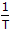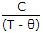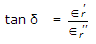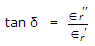# Electronics and Communication Engineering - Materials and Components

### Exercise :: Materials and Components - Section 1

31.

For a paramagnetic material, susceptiblity increases with increasing temperature.

 A. True B. False

Explanation:

Susceptibility ∝.

32.

Hydrogen is used in

 A. large size transformers B. MCB C. large size generators D. circuit breakers

Explanation:

Modem large size generators have hydrogen cooling.

33.

Assertion (A): Ferroelectric materials have spontaneous polarization.

Reason (R): Above curie temperature, ∈ =for ferro-electric materials.

 A. Both A and R are true and R is correct explanation of A B. Both A and R are true but R is not correct explanation of A C. A is true but R is false D. A is false but R is true

Explanation:

Both A and R are correct. Spontaneous polarization vanishes above ferroelectric curie temperature.

34.

If e is the charge of an electron, R is the radius of its orbit and ω is the angular velocity of electron, the magnetic dipole moment μm of the orbit is |μm| = eωR2.

 A. True B. False

Explanation:

1 μm 1 = 0.5 eωr2.

35.

If ∈r' and ∈r" are the real and imaginary parts of complex dielectric constant ∈r, and δ is the loss angle. Then

 A.B.C. tan δ = ∈'∈" D. tan δ = 1 - ∈'